()amax## ()amax

بازدید : 652 چهارشنبه 10 دي 1393

## amax()

This function returns an area maximum -- the maximum value in a grid spanning an X-Y region. The syntax is:

`amax(expr, xdim1, xdim2, ydim1, ydim2)`

where:

`expr    `any valid grads grid expression
``` xdim1   starting X or LON dimension expression  ``` ``` xdim2   ending X or LON dimension expression  ``` ``` ydim1   starting Y or LAT dimension expression  ``` ``` ydim2   ending Y or LAT dimension expression  ```

For global maximum, a shorthand may be used:

`amax(expr, global)` or
``` amax(expr, g) ```
is the same as
`amax(expr, lon=0, lon=360, lat=-90, lat=90)`

### Usage Notes

1. This function will only work with grads version 2.0.2 or later.
2. This function is more efficient that using nested `max` functions.
3. Related functions `amaxlocx` and `amaxlocy` will return the grid location (X or Y) of the maximum value. If more than one grid box contains the maximum value, the location returned will be the first one encountered as the grid is scanned. The grid is scanned by rows from south to north, and each row is scanned from west to east.
4. A similar set of functions exists for finding the minimum over an area: `amin`, `aminlocx`, and `aminlocy`.

### Examples

1. Get the maximum value of the variable `ps` over a specified grid domain:
``` d amax(ps,x=10,x=120,y=15,y=45) ```

2. Get the maximum value of the variable `sstanom` over the nino3.4 domain:
``` d amax(sstanom,lon=-170,lon=-120,lat=-5,lat=5) ```

3. Get the maximum value of the variable `slp` over the global domain, and also get the grid location of that minimum. Check results.
```
ga-> d amax(slp,g)

Result value = 105732

ga-> d amaxlocx(slp,g)

ga-> d amaxlocy(slp,g)

Result value = 168
Result value = 13

ga-> set y 13

ga-> set x 168
LON set to 83.5 83.5

LAT set to -84 -84
ga-> d slp
Result value = 105732

```
مطالب مرتبط
ارسال نظر برای این مطلب

نام
ایمیل (منتشر نمی‌شود) (لازم)
وبسایت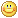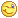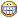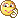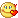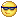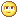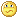نظر خصوصی
مشخصات شما ذخیره شود ؟ [حذف مشخصات] [شکلک ها]کد امنیتی
اطلاعات کاربری
 نام کاربری : رمز عبور :
• فراموشی رمز عبور؟
• لینک دوستان
آرشیو
آمار سایت
• کل مطالب : 155
• کل نظرات : 0
• افراد آنلاین : 3
• تعداد اعضا : 4
• آی پی امروز : 20
• آی پی دیروز : 19
• بازدید امروز : 76
• باردید دیروز : 268
• گوگل امروز : 1
• گوگل دیروز : 0
• بازدید هفته : 394
• بازدید ماه : 1,467
• بازدید سال : 35,872
• بازدید کلی : 156,924
• کدهای اختصاصی